Processing ......FreeComputerBooks.com Free Computer, Mathematics, Technical Books and Lecture Notes, etc.

Geometry and Topology
Related Book Categories:
•Euclidean Plane and its Relatives: A Minimalist Introduction

The book is designed for a semester-long course in Foundations of Geometry and meant to be rigorous, conservative, elementary and minimalist. It promotes the art and the skills of developing logical proofs.

•Elementary College Geometry (Henry Africk)

This text is intended for a brief introductory course in plane geometry, covers the topics from elementary geometry that are most likely to be required for more advanced mathematics courses. The only prerequisite is a semester of algebra.

•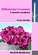Differential Geometry: A Geometric Introduction

This is the only book that introduces differential geometry through a combination of an intuitive geometric foundation, a rigorous connection with the standard formalisms, computer exercises with Maple, and a problems-based approach.

•Advances in Discrete Differential Geometry (Alexander I. Bobenko)

It surveys the fascinating connections between discrete models in differential geometry and complex analysis, integrable systems and applications in computer graphics and is illustrated to convince readers it's both beautiful and useful.

•Manifolds - Current Research Areas (Paul Bracken)

This book cover a number of subjects which will be of interest to workers in these areas. It is hoped that the papers here will be able to provide a useful resource for researchers with regard to current fields of research in this important area.

•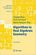Algorithms in Real Algebraic Geometry (Saugata Basu, et al)

The monograph gives a self-contained detailed exposition of the algorithmic real algebraic geometry. It will be useful both for beginners and for advanced readers, who work in real algebraic geometry or apply its methods in other fields.

•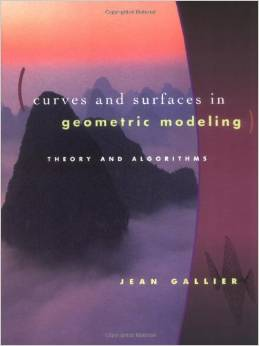Curves and Surfaces in Geometric Modeling: Theory and Algorithms

This book offers both a theoretically unifying understanding of polynomial curves and surfaces and an effective approach to implementation. It is also an exellent introduction to geometry concepts used in computer graphics, vision, robotics, geometric modeling.

•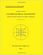Combinatorial Geometry with Application to Field Theory

Topics covered in this book include fundamental of combinatorics, algebraic combinatorics, topology with Smarandache geometry, combinatorial differential geometry, combinatorial Riemannian submanifolds, Lie multi-groups, etc.

•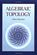Algebraic Topology (Allen Hatcher)

This introductory text is suitable for use in a course on the subject or for self-study, featuring broad coverage and a readable exposition, with many examples and exercises. The four main chapters present the basics: fundamental group and covering spaces, homology and cohomology, higher homotopy groups, and homotopy theory generally.

•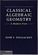Classical Algebraic Geometry: A Modern View (Igor V. Dolgachev)

This detailed exposition makes the rich legacy of classical algebraic geometry accessible to modern algebraic geometers and to others who are interested in applying classical results. Topics include plane algebraic curves of low degree, etc.

•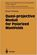Quasi-projective Moduli for Polarized Manifolds (Eckart Viehweg)

This book discusses two subjects of quite different nature: Construction methods for quotients of quasi-projective schemes by group actions or by equivalence relations and properties of direct images of certain sheaves under smooth morphisms.

•Convex Bodies and Algebraic Geometry: Theory of Toric Varieties

This book is an accessible introduction to current algebraic geometry of toric varieties gives new insight into continued fractions as well as their higher-dimensional analogues, the isoperimetric problem and other questions on convex bodies.

•Orbital Integrals on Reductive Lie Groups and Their Algebras

This book is to present a complete course on global analysis topics and establish some orbital applications of the integration on topological groups and their algebras to harmonic analysis and induced representations in representation theory.

•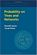Probability on Trees and Networks (Russell Lyons, et al)

This book is concerned with certain aspects of discrete probability on infinite graphs that are currently in vigorous development. Of course, finite graphs are analyzed as well, but usually with the aim of understanding infinite graphs and networks.

•Art Gallery Theorems and Algorithms (Joseph O'Rourke)

This book is the most comprehensive collection of results on polygons currently available and thus earns its place as a standard text in discrete and computational geometry. It is very well written and a pleasure to read.

•Shape Interrogation for Computer Aided Design and Manufacturing

This book provides the mathematical fundamentals as well as algorithms for various shape interrogation methods including nonlinear polynomial solvers, intersection problems, differential geometry of intersection curves, distance functions etc.

•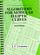Algorithms for Modular Elliptic Curves, 2nd Edition (J. E. Cremona)

This book presents a thorough treatment of many algorithms concerning the arithmetic of elliptic curves, with remarks on computer implementation. An extensive set of tables is provided giving the results of the author's implementation of the algorithms.

•Combinatorial and Computational Geometry (Jacob E. Goodman)

It includes surveys and research articles exploring geometric arrangements, polytopes, packing, covering, discrete convexity, geometric algorithms and their complexity, and the combinatorial complexity of geometric objects, particularly in low dimension.

•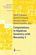Computations in Algebraic Geometry with Macaulay 2 (D.Eisenbud)

This book presents algorithmic tools for algebraic geometry and experimental applications of them using Macaulay 2.

•Strange Attractors: Creating Patterns in Chaos (Julien C. Sprott)

This book describes a simple method for generating an endless succession of beautiful fractal patterns by iterating simple maps and ordinary differential equations with coefficients chosen automatically by the computer.

•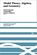Model Theory, Algebra, and Geometry (Deirdre Haskell, et al)

The book begins with an introduction to model theory. It then broadens into three components: pure model theory, geometry, and the model theory of fields.

•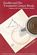Euclid and His Twentieth Century Rivals (Nathaniel Miller)

Twentieth-century developments in logic and mathematics have led many people to view Euclid's proofs as inherently informal, especially due to the use of diagrams in proofs. It introduces a diagrammatic computer proof system, based on this formal system.

•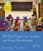3D Math Primer for Graphics and Game Development (F. Dunn)

Working C++ classes for mathematical and geometric entities and several different matrix classes illustrate how to put the techniques into practice, and exercises at the end of each chapter help reinforce the 3D programming concepts.

•Vector Math for 3D Computer Graphics, 4th Revision (Bradley Kjell)

This is a tutorial on vector algebra and matrix algebra from the viewpoint of computer graphics. It covers most vector and matrix topics needed to read college-level computer graphics text books.

•Mathematical Illustrations: A Manual of Geometry and PostScript

This practical introduction to the techniques needed to produce high-quality mathematical illustrations is suitable for anyone with basic knowledge of coordinate geometry.

•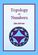Topology of Numbers (Allen Hatcher)

A textbook on elementary number theory from a geometric point of view, as opposed to the usual strictly algebraic approach. A fair amount of the book is devoted to studying Conway's topographs associated to quadratic forms in two variables.

•LEDA: A Platform for Combinatorial and Geometric Computing

LEDA is a library of efficient data types and algorithms and a platform for combinatorial and geometric computing. This book, written by the main authors of LEDA, is the definitive account of how the system operates and how it can be used.

•Algorithms and Data Structures: Apps to Graphics and Geometry

An introductory coverage of algorithms and data structures with application to graphics and geometry. Sample exercises, many with solutions, are included throughout the book.

•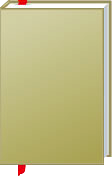Geometry and Topology

This is the previous page of Geometry and Topology, we are in the processing to convert all the books there to the new page. Please check this page again!!!

Book CategoriesAll CategoriesRecent BooksIT Research LibraryMiscellaneous Books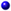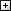Computer LanguagesComputer ScienceData Science/DatabasesElectronic EngineeringJava and Java EE (J2EE)Linux and UnixMathematicsMicrosoft and .NETMobile ComputingNetworking and CommunicationsSoftware EngineeringSpecial TopicsWeb Programming
Other Categories